# sklearn.gaussian_process.GaussianProcessRegressor¶

class sklearn.gaussian_process.GaussianProcessRegressor(kernel=None, *, alpha=1e-10, optimizer='fmin_l_bfgs_b', n_restarts_optimizer=0, normalize_y=False, copy_X_train=True, n_targets=None, random_state=None)[source]

Gaussian process regression (GPR).

The implementation is based on Algorithm 2.1 of [RW2006].

In addition to standard scikit-learn estimator API, GaussianProcessRegressor:

• allows prediction without prior fitting (based on the GP prior)

• provides an additional method sample_y(X), which evaluates samples drawn from the GPR (prior or posterior) at given inputs

• exposes a method log_marginal_likelihood(theta), which can be used externally for other ways of selecting hyperparameters, e.g., via Markov chain Monte Carlo.

To learn the difference between a point-estimate approach vs. a more Bayesian modelling approach, refer to the example entitled Comparison of kernel ridge and Gaussian process regression.

Read more in the User Guide.

New in version 0.18.

Parameters:
kernelkernel instance, default=None

The kernel specifying the covariance function of the GP. If None is passed, the kernel ConstantKernel(1.0, constant_value_bounds="fixed") * RBF(1.0, length_scale_bounds="fixed") is used as default. Note that the kernel hyperparameters are optimized during fitting unless the bounds are marked as “fixed”.

alphafloat or ndarray of shape (n_samples,), default=1e-10

Value added to the diagonal of the kernel matrix during fitting. This can prevent a potential numerical issue during fitting, by ensuring that the calculated values form a positive definite matrix. It can also be interpreted as the variance of additional Gaussian measurement noise on the training observations. Note that this is different from using a WhiteKernel. If an array is passed, it must have the same number of entries as the data used for fitting and is used as datapoint-dependent noise level. Allowing to specify the noise level directly as a parameter is mainly for convenience and for consistency with Ridge.

optimizer“fmin_l_bfgs_b”, callable or None, default=”fmin_l_bfgs_b”

Can either be one of the internally supported optimizers for optimizing the kernel’s parameters, specified by a string, or an externally defined optimizer passed as a callable. If a callable is passed, it must have the signature:

def optimizer(obj_func, initial_theta, bounds):
# * 'obj_func': the objective function to be minimized, which
#   takes the hyperparameters theta as a parameter and an
#   optional flag eval_gradient, which determines if the
# * 'initial_theta': the initial value for theta, which can be
#   used by local optimizers
# * 'bounds': the bounds on the values of theta
....
# Returned are the best found hyperparameters theta and
# the corresponding value of the target function.
return theta_opt, func_min


Per default, the L-BFGS-B algorithm from scipy.optimize.minimize is used. If None is passed, the kernel’s parameters are kept fixed. Available internal optimizers are: {'fmin_l_bfgs_b'}.

n_restarts_optimizerint, default=0

The number of restarts of the optimizer for finding the kernel’s parameters which maximize the log-marginal likelihood. The first run of the optimizer is performed from the kernel’s initial parameters, the remaining ones (if any) from thetas sampled log-uniform randomly from the space of allowed theta-values. If greater than 0, all bounds must be finite. Note that n_restarts_optimizer == 0 implies that one run is performed.

normalize_ybool, default=False

Whether or not to normalize the target values y by removing the mean and scaling to unit-variance. This is recommended for cases where zero-mean, unit-variance priors are used. Note that, in this implementation, the normalisation is reversed before the GP predictions are reported.

Changed in version 0.23.

copy_X_trainbool, default=True

If True, a persistent copy of the training data is stored in the object. Otherwise, just a reference to the training data is stored, which might cause predictions to change if the data is modified externally.

n_targetsint, default=None

The number of dimensions of the target values. Used to decide the number of outputs when sampling from the prior distributions (i.e. calling sample_y before fit). This parameter is ignored once fit has been called.

New in version 1.3.

random_stateint, RandomState instance or None, default=None

Determines random number generation used to initialize the centers. Pass an int for reproducible results across multiple function calls. See Glossary.

Attributes:
X_train_array-like of shape (n_samples, n_features) or list of object

Feature vectors or other representations of training data (also required for prediction).

y_train_array-like of shape (n_samples,) or (n_samples, n_targets)

Target values in training data (also required for prediction).

kernel_kernel instance

The kernel used for prediction. The structure of the kernel is the same as the one passed as parameter but with optimized hyperparameters.

L_array-like of shape (n_samples, n_samples)

Lower-triangular Cholesky decomposition of the kernel in X_train_.

alpha_array-like of shape (n_samples,)

Dual coefficients of training data points in kernel space.

log_marginal_likelihood_value_float

The log-marginal-likelihood of self.kernel_.theta.

n_features_in_int

Number of features seen during fit.

New in version 0.24.

feature_names_in_ndarray of shape (n_features_in_,)

Names of features seen during fit. Defined only when X has feature names that are all strings.

New in version 1.0.

GaussianProcessClassifier

Gaussian process classification (GPC) based on Laplace approximation.

References

Examples

>>> from sklearn.datasets import make_friedman2
>>> from sklearn.gaussian_process import GaussianProcessRegressor
>>> from sklearn.gaussian_process.kernels import DotProduct, WhiteKernel
>>> X, y = make_friedman2(n_samples=500, noise=0, random_state=0)
>>> kernel = DotProduct() + WhiteKernel()
>>> gpr = GaussianProcessRegressor(kernel=kernel,
...         random_state=0).fit(X, y)
>>> gpr.score(X, y)
0.3680...
>>> gpr.predict(X[:2,:], return_std=True)
(array([653.0..., 592.1...]), array([316.6..., 316.6...]))


Methods

 fit(X, y) Fit Gaussian process regression model. Get metadata routing of this object. get_params([deep]) Get parameters for this estimator. log_marginal_likelihood([theta, ...]) Return log-marginal likelihood of theta for training data. predict(X[, return_std, return_cov]) Predict using the Gaussian process regression model. sample_y(X[, n_samples, random_state]) Draw samples from Gaussian process and evaluate at X. score(X, y[, sample_weight]) Return the coefficient of determination of the prediction. set_params(**params) Set the parameters of this estimator. set_predict_request(*[, return_cov, return_std]) Request metadata passed to the predict method. set_score_request(*[, sample_weight]) Request metadata passed to the score method.
fit(X, y)[source]

Fit Gaussian process regression model.

Parameters:
Xarray-like of shape (n_samples, n_features) or list of object

Feature vectors or other representations of training data.

yarray-like of shape (n_samples,) or (n_samples, n_targets)

Target values.

Returns:
selfobject

GaussianProcessRegressor class instance.

Get metadata routing of this object.

Please check User Guide on how the routing mechanism works.

Returns:

A MetadataRequest encapsulating routing information.

get_params(deep=True)[source]

Get parameters for this estimator.

Parameters:
deepbool, default=True

If True, will return the parameters for this estimator and contained subobjects that are estimators.

Returns:
paramsdict

Parameter names mapped to their values.

Return log-marginal likelihood of theta for training data.

Parameters:
thetaarray-like of shape (n_kernel_params,) default=None

Kernel hyperparameters for which the log-marginal likelihood is evaluated. If None, the precomputed log_marginal_likelihood of self.kernel_.theta is returned.

If True, the gradient of the log-marginal likelihood with respect to the kernel hyperparameters at position theta is returned additionally. If True, theta must not be None.

clone_kernelbool, default=True

If True, the kernel attribute is copied. If False, the kernel attribute is modified, but may result in a performance improvement.

Returns:
log_likelihoodfloat

Log-marginal likelihood of theta for training data.

Gradient of the log-marginal likelihood with respect to the kernel hyperparameters at position theta. Only returned when eval_gradient is True.

predict(X, return_std=False, return_cov=False)[source]

Predict using the Gaussian process regression model.

We can also predict based on an unfitted model by using the GP prior. In addition to the mean of the predictive distribution, optionally also returns its standard deviation (return_std=True) or covariance (return_cov=True). Note that at most one of the two can be requested.

Parameters:
Xarray-like of shape (n_samples, n_features) or list of object

Query points where the GP is evaluated.

return_stdbool, default=False

If True, the standard-deviation of the predictive distribution at the query points is returned along with the mean.

return_covbool, default=False

If True, the covariance of the joint predictive distribution at the query points is returned along with the mean.

Returns:
y_meanndarray of shape (n_samples,) or (n_samples, n_targets)

Mean of predictive distribution a query points.

y_stdndarray of shape (n_samples,) or (n_samples, n_targets), optional

Standard deviation of predictive distribution at query points. Only returned when return_std is True.

y_covndarray of shape (n_samples, n_samples) or (n_samples, n_samples, n_targets), optional

Covariance of joint predictive distribution a query points. Only returned when return_cov is True.

sample_y(X, n_samples=1, random_state=0)[source]

Draw samples from Gaussian process and evaluate at X.

Parameters:
Xarray-like of shape (n_samples_X, n_features) or list of object

Query points where the GP is evaluated.

n_samplesint, default=1

Number of samples drawn from the Gaussian process per query point.

random_stateint, RandomState instance or None, default=0

Determines random number generation to randomly draw samples. Pass an int for reproducible results across multiple function calls. See Glossary.

Returns:
y_samplesndarray of shape (n_samples_X, n_samples), or (n_samples_X, n_targets, n_samples)

Values of n_samples samples drawn from Gaussian process and evaluated at query points.

score(X, y, sample_weight=None)[source]

Return the coefficient of determination of the prediction.

The coefficient of determination $$R^2$$ is defined as $$(1 - \frac{u}{v})$$, where $$u$$ is the residual sum of squares ((y_true - y_pred)** 2).sum() and $$v$$ is the total sum of squares ((y_true - y_true.mean()) ** 2).sum(). The best possible score is 1.0 and it can be negative (because the model can be arbitrarily worse). A constant model that always predicts the expected value of y, disregarding the input features, would get a $$R^2$$ score of 0.0.

Parameters:
Xarray-like of shape (n_samples, n_features)

Test samples. For some estimators this may be a precomputed kernel matrix or a list of generic objects instead with shape (n_samples, n_samples_fitted), where n_samples_fitted is the number of samples used in the fitting for the estimator.

yarray-like of shape (n_samples,) or (n_samples, n_outputs)

True values for X.

sample_weightarray-like of shape (n_samples,), default=None

Sample weights.

Returns:
scorefloat

$$R^2$$ of self.predict(X) w.r.t. y.

Notes

The $$R^2$$ score used when calling score on a regressor uses multioutput='uniform_average' from version 0.23 to keep consistent with default value of r2_score. This influences the score method of all the multioutput regressors (except for MultiOutputRegressor).

set_params(**params)[source]

Set the parameters of this estimator.

The method works on simple estimators as well as on nested objects (such as Pipeline). The latter have parameters of the form <component>__<parameter> so that it’s possible to update each component of a nested object.

Parameters:
**paramsdict

Estimator parameters.

Returns:
selfestimator instance

Estimator instance.

set_predict_request(*, return_cov: Union[bool, None, str] = '$UNCHANGED$', return_std: Union[bool, None, str] = '$UNCHANGED$') [source]

Request metadata passed to the predict method.

Note that this method is only relevant if enable_metadata_routing=True (see sklearn.set_config). Please see User Guide on how the routing mechanism works.

The options for each parameter are:

• True: metadata is requested, and passed to predict if provided. The request is ignored if metadata is not provided.

• False: metadata is not requested and the meta-estimator will not pass it to predict.

• None: metadata is not requested, and the meta-estimator will raise an error if the user provides it.

• str: metadata should be passed to the meta-estimator with this given alias instead of the original name.

The default (sklearn.utils.metadata_routing.UNCHANGED) retains the existing request. This allows you to change the request for some parameters and not others.

New in version 1.3.

Note

This method is only relevant if this estimator is used as a sub-estimator of a meta-estimator, e.g. used inside a Pipeline. Otherwise it has no effect.

Parameters:
return_covstr, True, False, or None, default=sklearn.utils.metadata_routing.UNCHANGED

Metadata routing for return_cov parameter in predict.

return_stdstr, True, False, or None, default=sklearn.utils.metadata_routing.UNCHANGED

Metadata routing for return_std parameter in predict.

Returns:
selfobject

The updated object.

set_score_request(*, sample_weight: Union[bool, None, str] = '$UNCHANGED$') [source]

Request metadata passed to the score method.

Note that this method is only relevant if enable_metadata_routing=True (see sklearn.set_config). Please see User Guide on how the routing mechanism works.

The options for each parameter are:

• True: metadata is requested, and passed to score if provided. The request is ignored if metadata is not provided.

• False: metadata is not requested and the meta-estimator will not pass it to score.

• None: metadata is not requested, and the meta-estimator will raise an error if the user provides it.

• str: metadata should be passed to the meta-estimator with this given alias instead of the original name.

The default (sklearn.utils.metadata_routing.UNCHANGED) retains the existing request. This allows you to change the request for some parameters and not others.

New in version 1.3.

Note

This method is only relevant if this estimator is used as a sub-estimator of a meta-estimator, e.g. used inside a Pipeline. Otherwise it has no effect.

Parameters:
sample_weightstr, True, False, or None, default=sklearn.utils.metadata_routing.UNCHANGED

Metadata routing for sample_weight parameter in score.

Returns:
selfobject

The updated object.

## Examples using sklearn.gaussian_process.GaussianProcessRegressor¶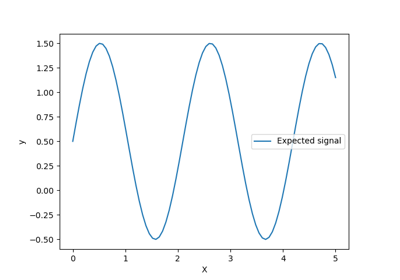Ability of Gaussian process regression (GPR) to estimate data noise-level

Ability of Gaussian process regression (GPR) to estimate data noise-level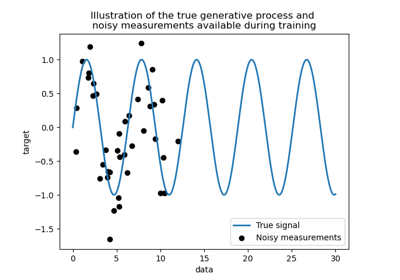Comparison of kernel ridge and Gaussian process regression

Comparison of kernel ridge and Gaussian process regression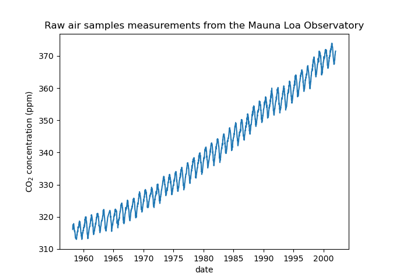Forecasting of CO2 level on Mona Loa dataset using Gaussian process regression (GPR)

Forecasting of CO2 level on Mona Loa dataset using Gaussian process regression (GPR)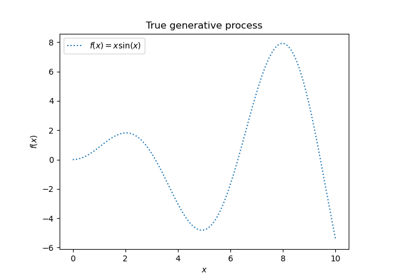Gaussian Processes regression: basic introductory example

Gaussian Processes regression: basic introductory example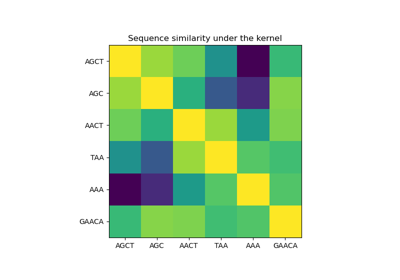Gaussian processes on discrete data structures

Gaussian processes on discrete data structures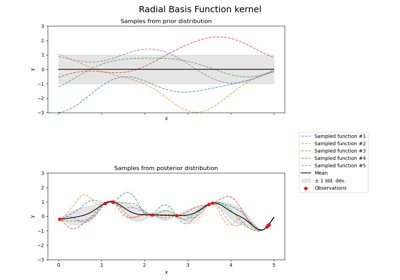Illustration of prior and posterior Gaussian process for different kernels

Illustration of prior and posterior Gaussian process for different kernels